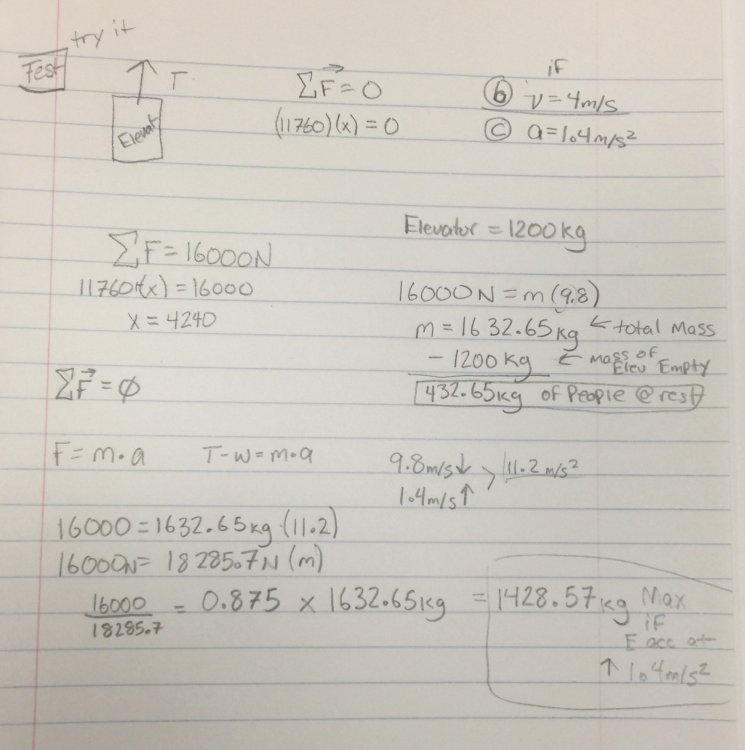# Just took a test can't figure out how to solve (Elevator Physics Problem)

## Recommended Posts

Ok so so far I have not struggled with the elevator problems however, the one on the test I was a little off in my method and couldn't get the answer.

The question is something like:

An elevator has a cable with the max force of 16,000N, the mass of the elevator is 1200kg (a)what is the maximum mass of the passengers if the elevator is at rest (this part I got, I think)? (b) What is the max capacity if the elevator is moving at a constant v of 4m/s (c) what is the max capacity if the elevator is accelerating up at 1.4m/s^2?

I got the same answer for (a) & (b) which was approximately 435kg

for (c) I got something like .85 which I then multiplied by the 1635kg I got for part a and b and got something ~1390kg or ~1400kg when the elevator is accelerating up.

What did I do wrong?

Edit: I replicated my work just now on a scrap paper. I just attached it I skipped (b) because I got the same answer when I did it in class so if I am wrong (which i'm at least 70% sure I am) I would like to know if someone can explain to me where I went wrong.Edited by FrankP

##### Share on other sites

So 1428.57 kg maximum on cable accelerating upward. Capacity would be that - the mass of the empty elevator or 228.57kg

##### Share on other sites
1 hour ago, J.C.MacSwell said:

So 1428.57 kg maximum on cable accelerating upward. Capacity would be that - the mass of the empty elevator or 228.57kg

Ok so I am going to basically work backwards with your numbers to try and rectify the math because I know I am going to need to be able to do this on my own going forward. Thank you again

##### Share on other sites

1200kg is (1200)(9.81)= 11772 N. The maximum force on the cable can be 16000 N which leaves 16000- 11772= 4228 N for the passengers.  That corresponds to 4228/9.81= 431 kg. That's close enough to your 435 kg.  We probably rounded differently.  You are correct that the elevator moving at a constant speed does not change that.  Accelerating upward at 1.4 m/s^2 adds an additional force of 1.4m where m is the mass.  The tension on the cable due to the elevator car itself is (1200)(9.81+ 1.4)= (1200)(11.21)= 1200= 13452 N.  That leaves 14000- 13452= 548 N for the passengers.  That, including the acceleration, is  549/(9.81+ 1.4)= 11.21 kg.

##### Share on other sites
2 hours ago, HallsofIvy said:

1200kg is (1200)(9.81)= 11772 N. The maximum force on the cable can be 16000 N which leaves 16000- 11772= 4228 N for the passengers.  That corresponds to 4228/9.81= 431 kg. That's close enough to your 435 kg.  We probably rounded differently.  You are correct that the elevator moving at a constant speed does not change that.  Accelerating upward at 1.4 m/s^2 adds an additional force of 1.4m where m is the mass.  The tension on the cable due to the elevator car itself is (1200)(9.81+ 1.4)= (1200)(11.21)= 1200= 13452 N.  That leaves 14000- 13452= 548 N for the passengers.  That, including the acceleration, is  549/(9.81+ 1.4)= 11.21 kg.

Except 16000 is allowable as you used initially, not 14000 (which would have allowed about 49kg not 11.21 by your method, which is sound otherwise, but with 16000 it is around 228 kg)

Otherwise the difference was just that you used 9.81 for g and FrankP used 9.8

Edited by J.C.MacSwell

##### Share on other sites

Interesting how you worked out the problem i’m going to have to try this again tomorrow now that I saw the comments! Thanks for the help I wish I had a good example like this to work on before taking the test lol

##### Share on other sites
19 hours ago, J.C.MacSwell said:

Except 16000 is allowable as you used initially, not 14000 (which would have allowed about 49kg not 11.21 by your method, which is sound otherwise, but with 16000 it is around 228 kg)

Otherwise the difference was just that you used 9.81 for g and FrankP used 9.8

Ok so when I redid the problem today I realized that all along I had 228 as my answer I just never subtracted from the mass of the elevator. So technically the math checks out I guess I hope that is what I got on the test because now I can't remember but one can only hope... For the future though I do appreciate the help as I know this is only the beginning of elevator problems and more advanced vector based problems so I do appreciate it.

## Create an account

Register a new account